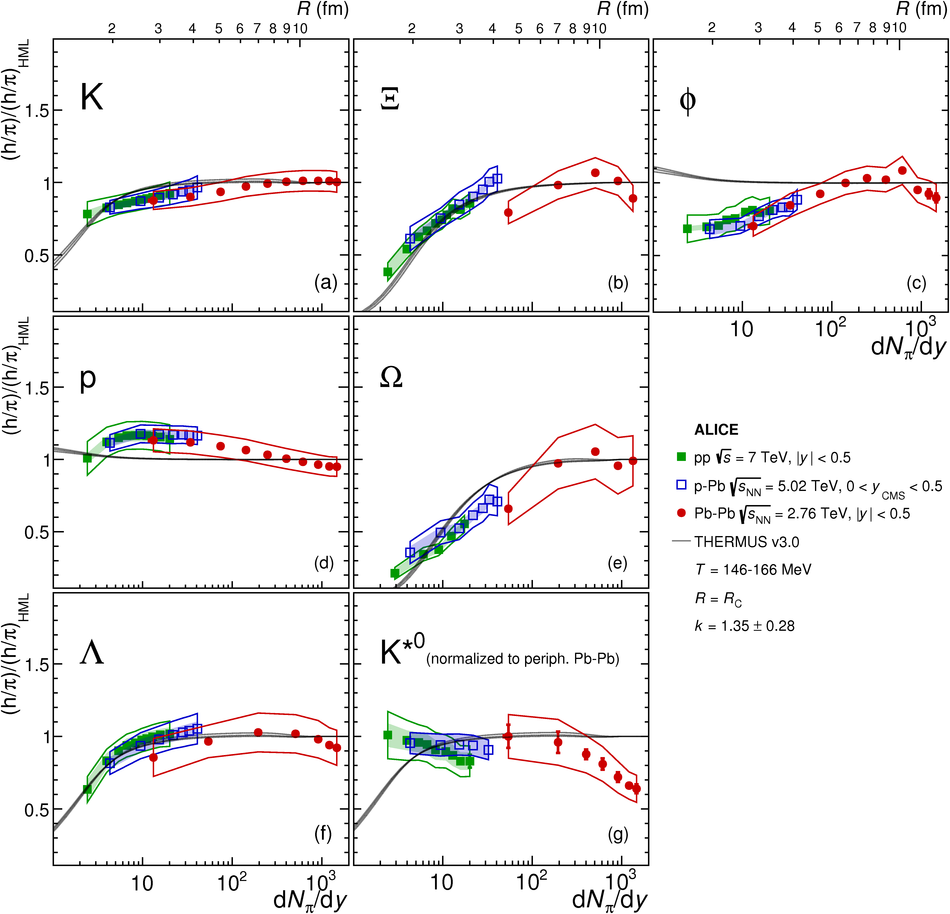Multiplicity dependence of light-flavor hadron production in pp collisions at $\sqrt{s}$ = 7 TeV

Comprehensive results on the production of unidentified charged particles, $\pi^{\pm}$, $\rm{K}^{\pm}$, $\rm{K}^{0}_{S}$, $\rm{K}$*(892)$^{0}$, $\rm{p}$, $\overline{\rm{p}}$, $\phi$(1020), $\Lambda$, $\overline{\Lambda}$, $\Xi^{-}$, $\overline{\Xi}^{+}$, $\Omega^{-}$ and $\overline{\Omega}^{+}$ hadrons in proton-proton (pp) collisions at $\sqrt{s}$ = 7 TeV at midrapidity ($|y| <~ 0.5$) as a function of charged-particle multiplicity density are presented. In order to avoid auto-correlation biases, the actual transverse momentum ($p_{\rm{T}}$) spectra of the particles under study and the event activity are measured in different rapidity windows. In the highest multiplicity class, the charged-particle density reaches about 3.5 times the value measured in inelastic collisions. While the yield of protons normalized to pions remains approximately constant as a function of multiplicity, the corresponding ratios of strange hadrons to pions show a significant enhancement that increases with increasing strangeness content. Furthermore, all identified particle to pion ratios are shown to depend solely on charged-particle multiplicity density, regardless of system type and collision energy. The evolution of the spectral shapes with multiplicity and hadron mass shows patterns that are similar to those observed in p-Pb and Pb-Pb collisions at LHC energies. The obtained $p_{\rm{T}}$ distributions and yields are compared to expectations from QCD-based pp event generators as well as to predictions from thermal and hydrodynamic models. These comparisons indicate that traces of a collective, equilibrated system are already present in high-multiplicity pp collisions.

Figures

Figure 1

 Multiplicity dependence of charged and neutral kaon yields obtained using (a) mid-pseudorapidity charged particle multiplicities ($|\eta|< 0.5$) and (b) the charged particle multiplicities within the pseudorapidity range corresponding to the V0A and V0C detectors (denoted by V0M, corresponding to $-3.7< \eta< -1.7$ and $2.8< \eta< 5.1$) in PYTHIA8 simulations of inelastic pp collisions at \seven.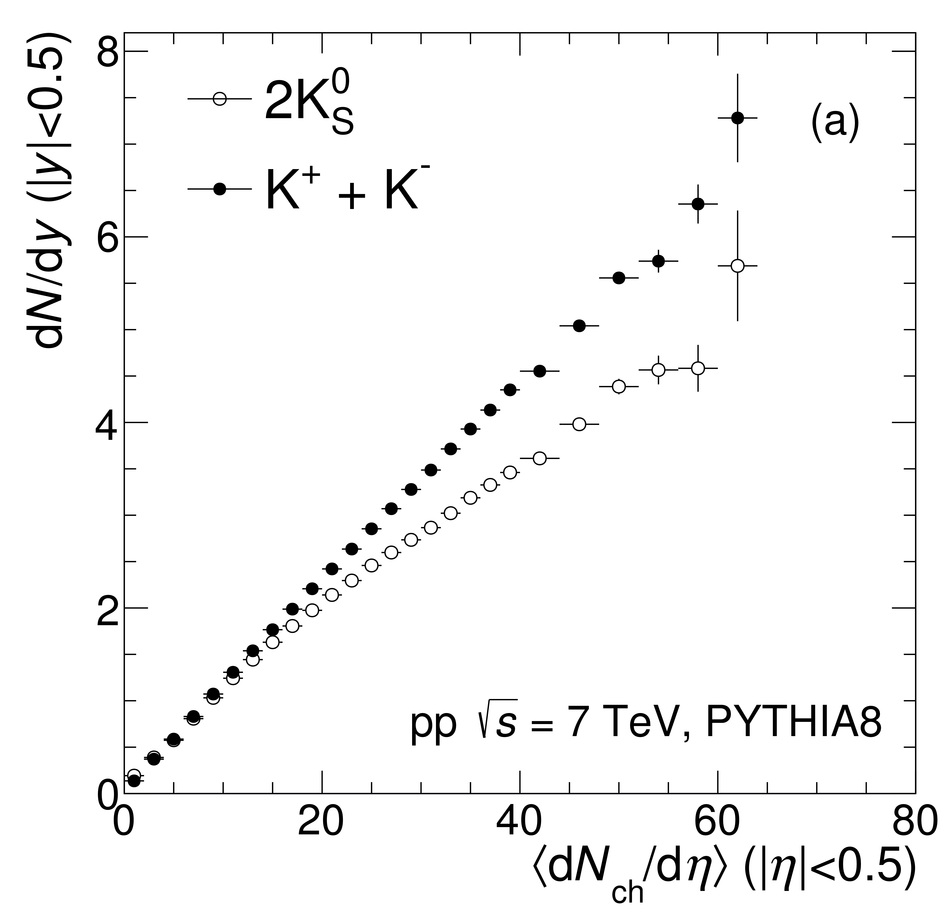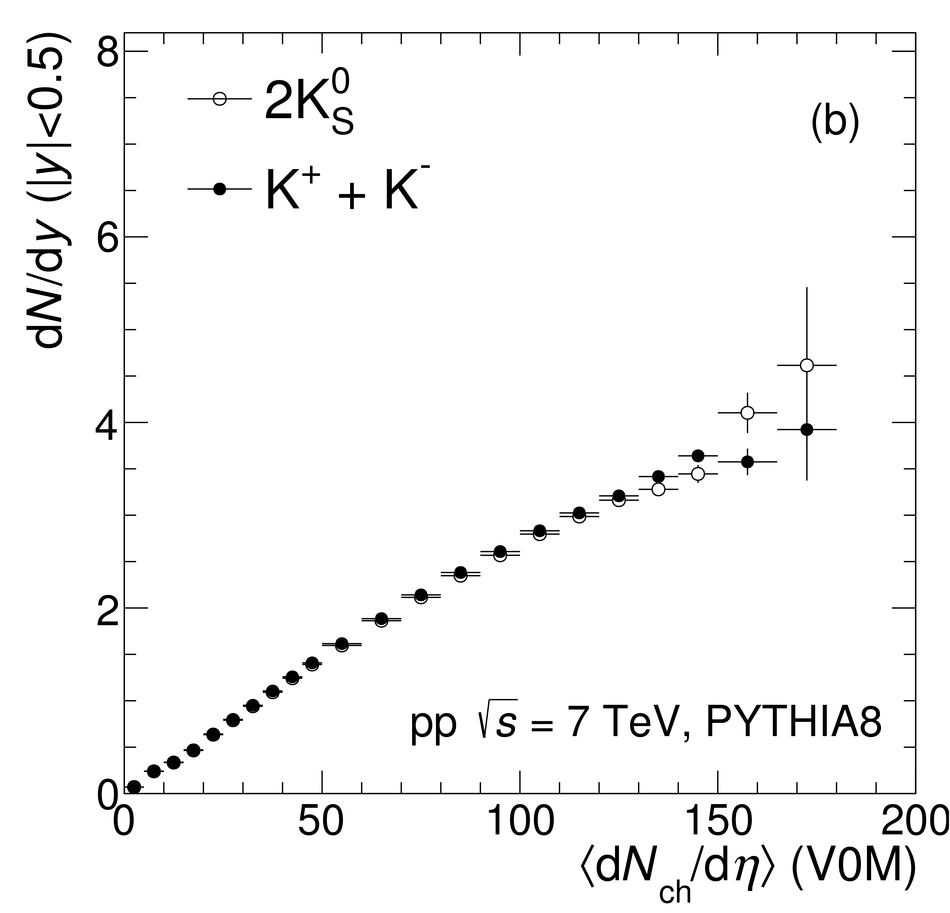Figure 2

 Transverse momentum spectra of the sum of positively and negatively charged particles in different V0M event multiplicity classesFigure 3

 Transverse momentum spectra of (a) \pipm, (b) \kapm, (c) \kzero, (d) \kstar, (e) \p+\pbar, (f) $\phi$, (g) $\lmb$+$\almb$, (h) \X+\Ix and (i) \Om+\Mo. Top to bottom: high to low multiplicity; data are scaled by $2^{n}$ factors for better visibility.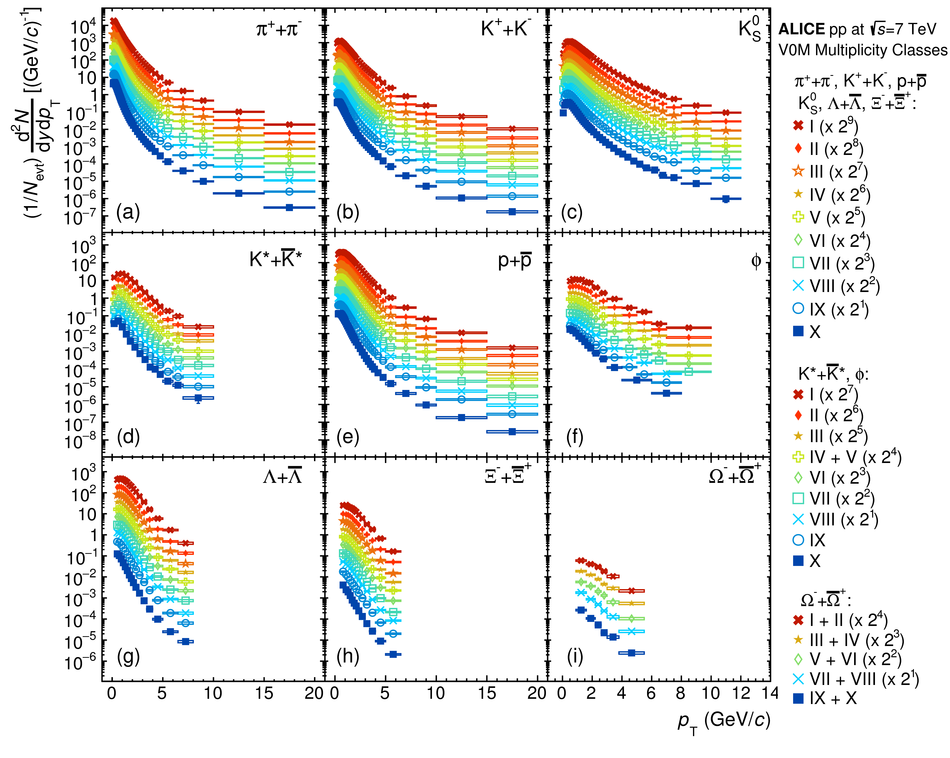Figure 4

 Unidentified and identified particle spectra modification in (a) high-multiplicity and (b) low-multiplicity event classes. Statistical uncertainties are shown as errors bars and systematic uncertainties that are uncorrelated across multiplicities are shown as boxes. Other uncertainties are disregarded as they cancel in the ratio.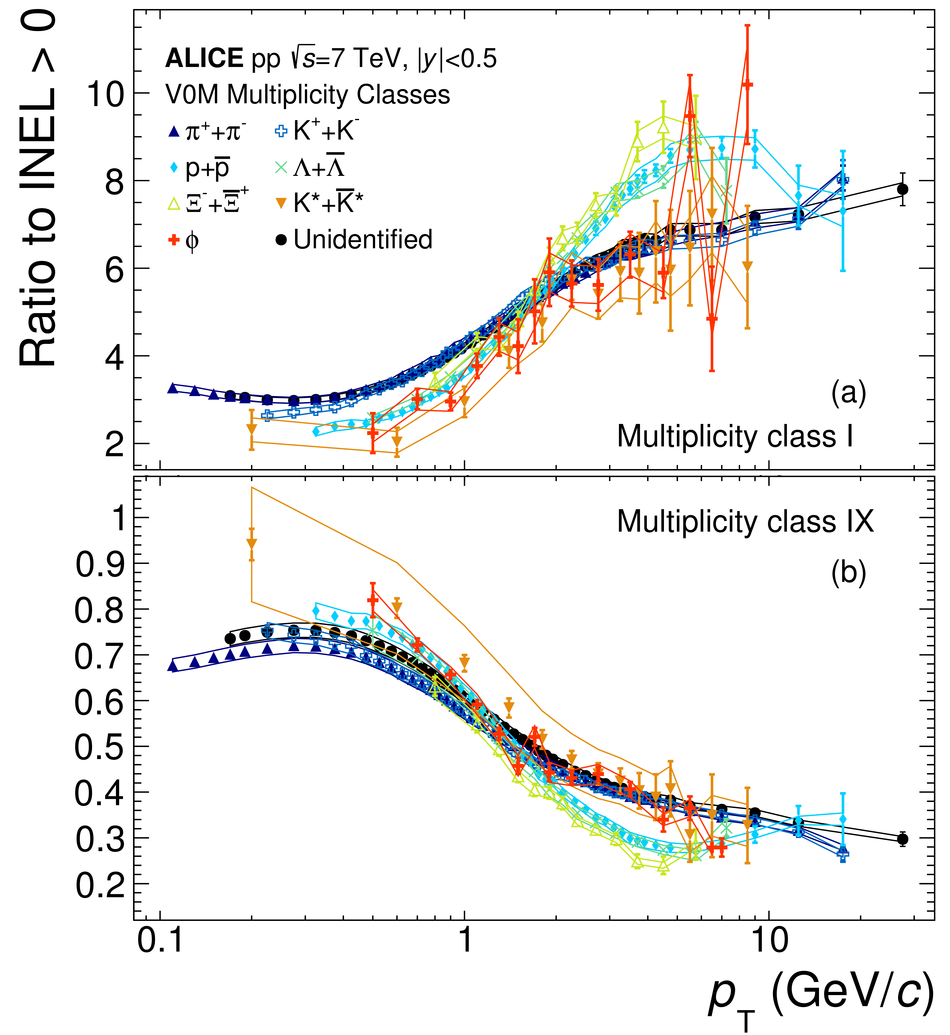Figure 5

 Transverse momentum dependence of (a, b, c) \p/$\phi$~=~(p + \pbar)/$\phi$, (d, e, f) \kpi~=~(\kap + \kam)/(\pip + \pim), (g, h, i) \ppi~=~(p + \pbar)/(\pip +\pim) and (j, k, l) \lmb/\kzero\ (from top to bottom row) yield ratios in (a, d, g, j) pp, (b, e, h, k) p-Pb and (c, f, i, l) Pb-Pb collisions for high- and low-multiplicity classes, respectively. Cancellation of common systematic uncertainties in the numerator and denominator was carried out only for the \lmb/\kzero, as in other cases the cancellation is non-trivial because of the use of various combined identification techniques or, in the case of resonances, of significantly different analysis strategy. Reference p-Pb and Pb-Pb data from .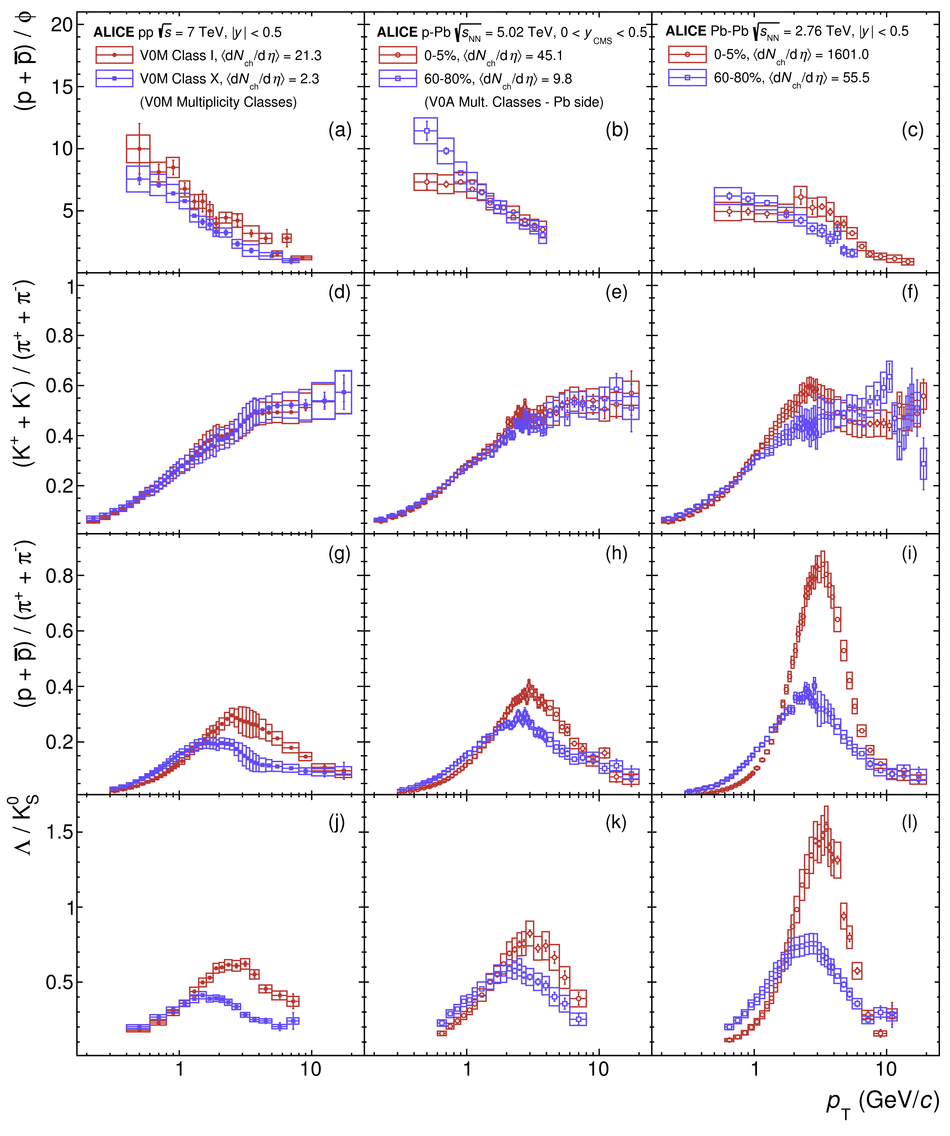Figure 6

 Transverse momentum dependence of (a, b, c) \p/$\phi$~=~(p + \pbar)/$\phi$, (d, e, f) \kpi~=~(\kap + \kam)/(\pip + \pim), (g, h, i) \ppi~=~(p + \pbar)/(\pip +\pim) and (j, k, l) \lmb/\kzero\ yield ratios in (a, d, g, j) low-, (b, e, h, k) mid- and (c, f, i, l) high-\pt\ intervals in pp, p-Pb and Pb-Pb collisions as a function of \avdNdeta. Reference p-Pb and Pb-Pb data from .Figure 7

 Average transverse momentum (a) \kstar\ (left) and (b) $\phi$ compared to protons as a function of charged-particle multiplicity density.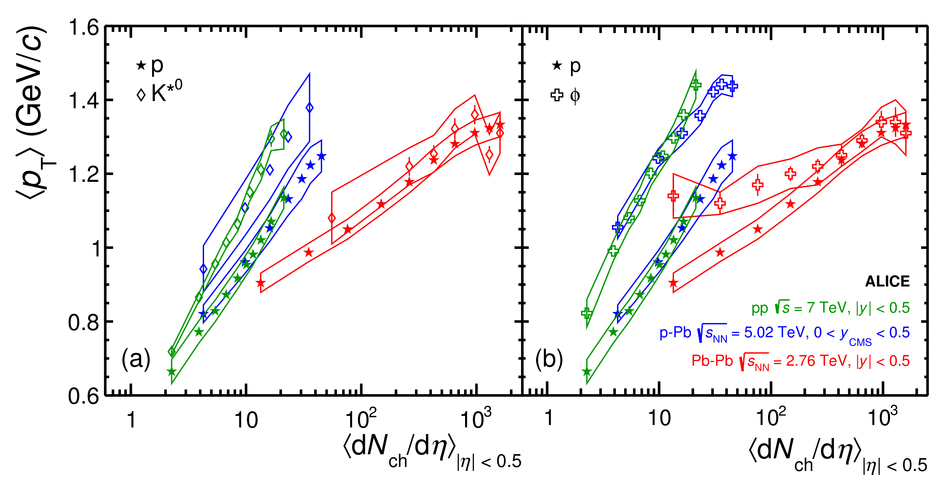Figure 8

 Integrated particle-to-pion ratios as a function of \avdNdeta\ for pp, p-Pb and Pb-Pb collisions. Reference p-Pb and Pb-Pb data from .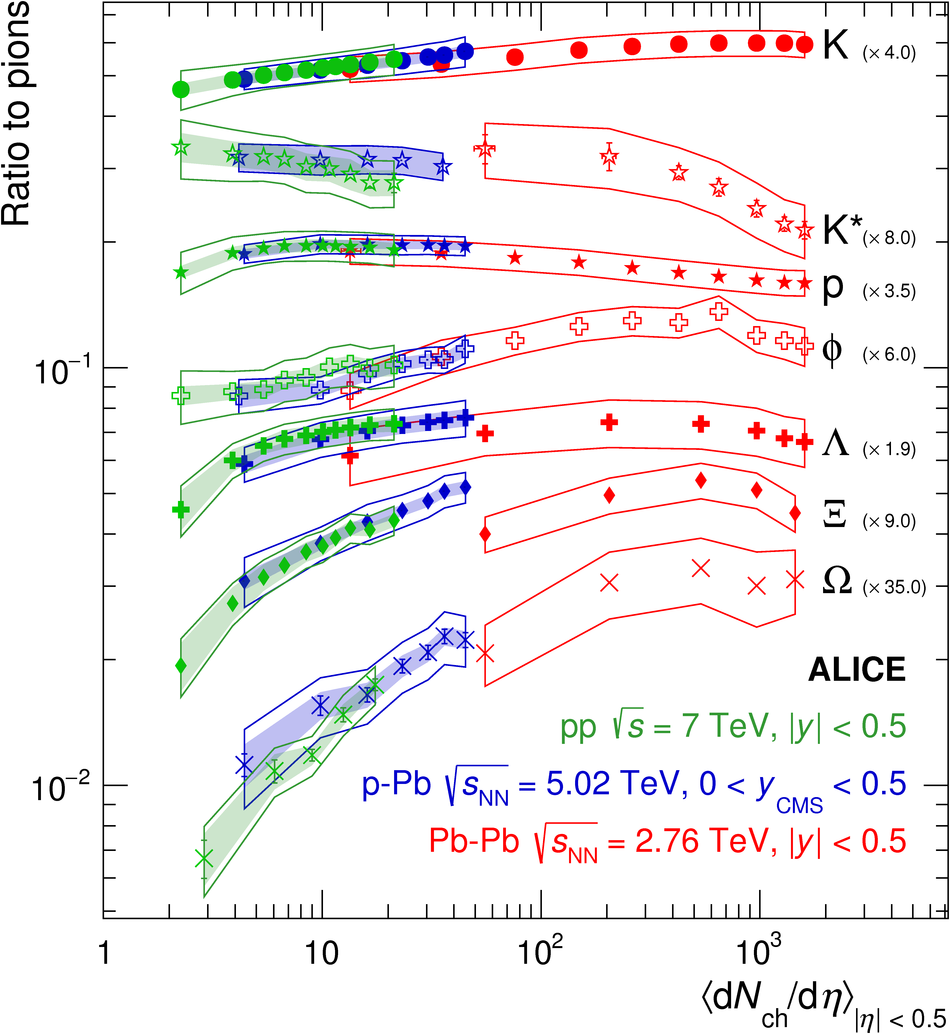Figure 9

 Transverse momentum dependence of (a, b, c) \p/$\phi$~=~(p + \pbar)/$\phi$, (d, e, f) \kpi~=~(\kap + \kam)/(\pip + \pim), (g, h, i) \ppi~=~(p + \pbar)/(\pip +\pim) and (j, k, l) \lmb/\kzero\ yield ratios in (a, d, g, j) low-, (b, e, h, k) mid- and (c, f, i, l) high-\pt\ intervals compared to several Monte Carlo event generators. Statistical uncertainties are shown as error bars, total systematic uncertainties are shown as hollow bands and multiplicity-uncorrelated systematic uncertainties are shown as shaded bands.Figure 10

 Integrated yield ratios as a function of charged-particle multiplicity density compared to several Monte Carlo event generators. Statistical uncertainties are shown as error bars, total systematic uncertainties are shown as hollow bands and multiplicity-uncorrelated systematic uncertainties are shown as shaded bands. For a complete description, please refer to the text.Figure 11

 (a) Simultaneous BG-BW fit to $\pi$, $K$ and \p\ spectra from high-multiplicity (I+II V0M classes) \pp\ collisions Solid lines correspond to the fit, dashed lines to the prediction. (b, c, d, e, f, g, h, i, j) Ratio data/fit(prediction) for the various particle species.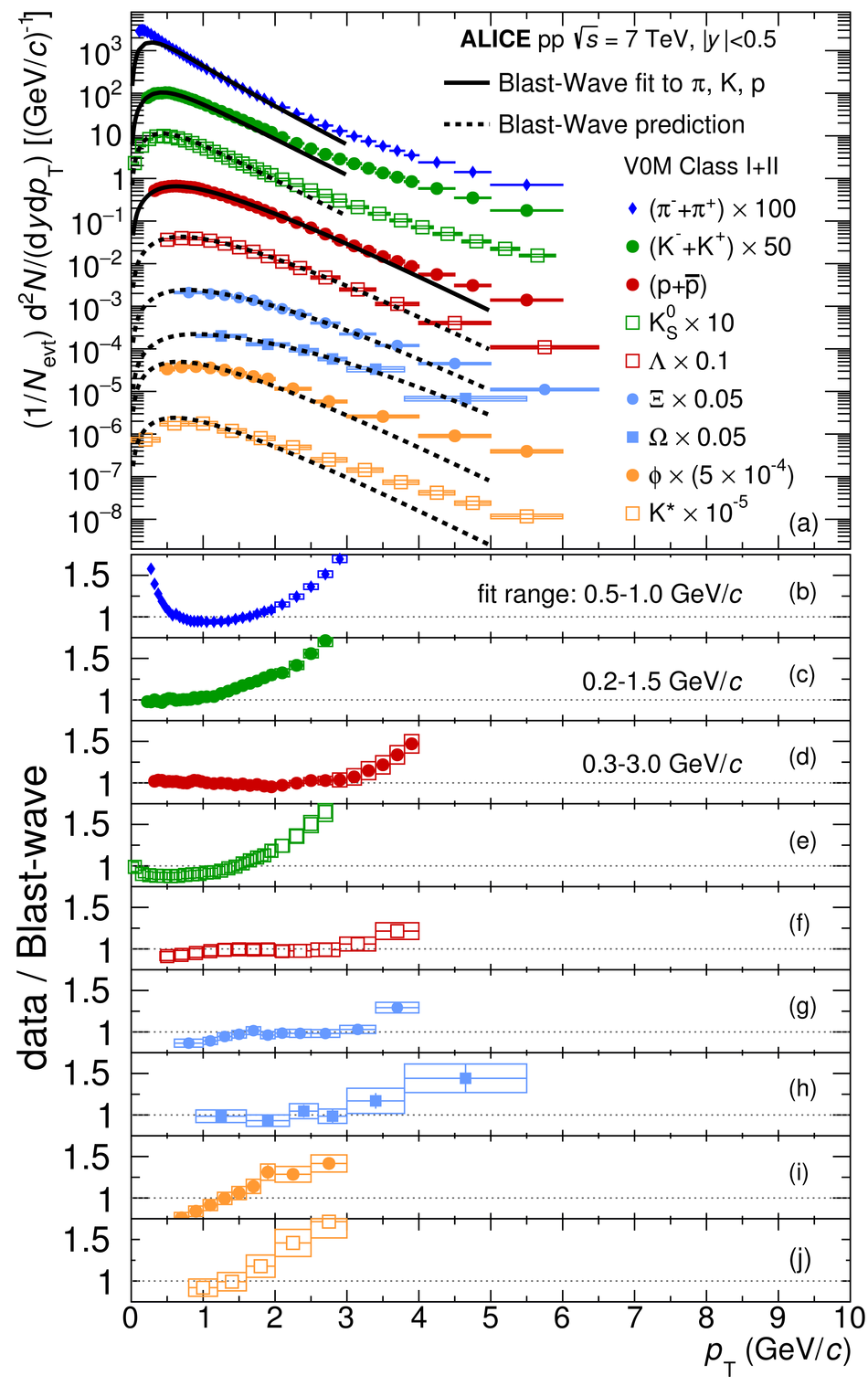Figure 12

 Kinematic freeze-out temperature parameter \Tkin\ versus average expansion velocity \betaT\ from a simultaneous BG-BW fit to $\pi$, $K$ and \p\ spectra measured in \pp, \pPb\ and \PbPb\ collisions. The shade of the datapoints indicates the corresponding average charged-particle multiplicity density.Figure 13

 Ratios of the production yields to pions for several particle species as a function of the midrapidity pion yields for pp, \pPb\ and \PbPb\ colliding systems compared to the THERMUS model prediction for the strangeness canonical suppression (continuous curves), in which only the system size is varied. All values except for the \kstar\ are normalized to the high multiplicity limit (see text for details). The upper axis shows the radius $R$ of the correlation volume $V=R^{3}$ which corresponds to the predicted particle ratios. The variations in model prediction curves correspond to variations of the chemical freeze-out temperature between 146, 156 and 166~MeV. Reference p-Pb and Pb-Pb data from